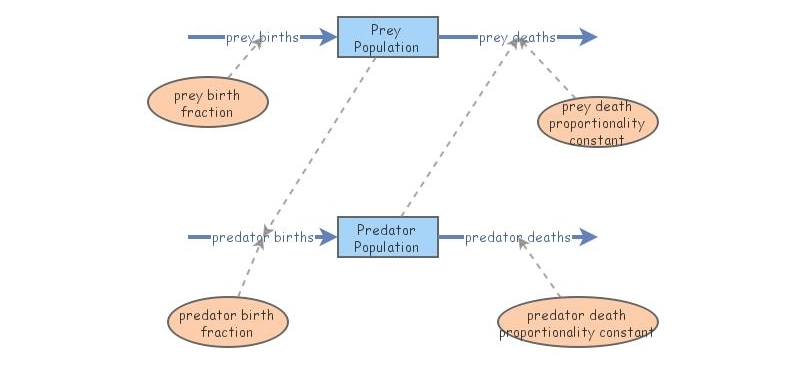Predator-Prey Model ("Lotka'Volterra")Dynamic simulation modelers are particularly interested in understanding and being able to distinguish between the behavior of stocks and flows that result from internal interactions and those that result from external forces acting on a system.  For some time modelers have been particularly interested in internal interactions that result in stable oscillations in the absence of any external forces acting on a system.  The model in this last scenario was independently developed by Alfred Lotka (1924) and Vito Volterra (1926).  Lotka was interested in understanding internal dynamics that might explain oscillations in moth and butterfly populations and the parasitoids that attack them.  Volterra was interested in explaining an increase in coastal populations of predatory fish and a decrease in their prey that was observed during World War I when human fishing pressures on the predator species declined.  Both discovered that a relatively simple model is capable of producing the cyclical behaviors they observed.  Since that time, several researchers have been able to reproduce the modeling dynamics in simple experimental systems consisting of only predators and prey.  It is now generally recognized that the model world that Lotka and Volterra produced is too simple to explain the complexity of most and predator-prey dynamics in nature.  And yet, the model significantly advanced our understanding of the critical role of feedback in predator-prey interactions and in feeding relationships that result in community dynamics.The Lotka–Volterra model makes a number of assumptions about the environment and evolution of the predator and prey populations:

1. The prey population finds ample food at all times.
2. The food supply of the predator population depends entirely on the size of the prey population.
3. The rate of change of population is proportional to its size.
4. During the process, the environment does not change in favour of one species and genetic adaptation is inconsequential.
5. Predators have limitless appetite.
As differential equations are used, the solution is deterministic and continuous. This, in turn, implies that the generations of both the predator and prey are continually overlapping.

Prey
When multiplied out, the prey equation becomes
dx/dtαx - βxy
The prey are assumed to have an unlimited food supply, and to reproduce exponentially unless subject to predation; this exponential growth is represented in the equation above by the term αx. The rate of predation upon the prey is assumed to be proportional to the rate at which the predators and the prey meet; this is represented above by βxy. If either x or y is zero then there can be no predation.

With these two terms the equation above can be interpreted as: the change in the prey's numbers is given by its own growth minus the rate at which it is preyed upon.

### Predators

The predator equation becomes

dy/dt =-In this equation,represents the growth of the predator population. (Note the similarity to the predation rate; however, a different constant is used as the rate at which the predator population grows is not necessarily equal to the rate at which it consumes the prey).represents the loss rate of the predators due to either natural death or emigration; it leads to an exponential decay in the absence of prey.

Hence the equation expresses the change in the predator population as growth fueled by the food supply, minus natural death.